JEE  >  JEE Main Mock - 3 (Previous Pattern)

# JEE Main Mock - 3 (Previous Pattern)

Test Description

## 90 Questions MCQ Test Mock Test Series for JEE Main & Advanced | JEE Main Mock - 3 (Previous Pattern)

JEE Main Mock - 3 (Previous Pattern) for JEE 2022 is part of Mock Test Series for JEE Main & Advanced preparation. The JEE Main Mock - 3 (Previous Pattern) questions and answers have been prepared according to the JEE exam syllabus.The JEE Main Mock - 3 (Previous Pattern) MCQs are made for JEE 2022 Exam. Find important definitions, questions, notes, meanings, examples, exercises, MCQs and online tests for JEE Main Mock - 3 (Previous Pattern) below.
Solutions of JEE Main Mock - 3 (Previous Pattern) questions in English are available as part of our Mock Test Series for JEE Main & Advanced for JEE & JEE Main Mock - 3 (Previous Pattern) solutions in Hindi for Mock Test Series for JEE Main & Advanced course. Download more important topics, notes, lectures and mock test series for JEE Exam by signing up for free. Attempt JEE Main Mock - 3 (Previous Pattern) | 90 questions in 180 minutes | Mock test for JEE preparation | Free important questions MCQ to study Mock Test Series for JEE Main & Advanced for JEE Exam | Download free PDF with solutions
 1 Crore+ students have signed up on EduRev. Have you?
JEE Main Mock - 3 (Previous Pattern) - Question 1

### The phase difference between the alternating current and e.m.f. is π/2. Which of the following can not be the constituent of the circuit?

JEE Main Mock - 3 (Previous Pattern) - Question 2

### The frequency for which a 5 μF capacitor has a reactance of 1/1000 ohm is given by

JEE Main Mock - 3 (Previous Pattern) - Question 3

### Neutrino is a particle, which is

JEE Main Mock - 3 (Previous Pattern) - Question 4

Which of the following is true?

JEE Main Mock - 3 (Previous Pattern) - Question 5

A car is moving in a circular horizontal track of radius 10 m with a constant speed of 10 m/sec. A plump bob is suspended from the roof of the car by a light rigid rod of length 1.00 m. The angle made by the rod with track is

JEE Main Mock - 3 (Previous Pattern) - Question 6

The approximated wavelength of a photon of energy 2.48eV is

JEE Main Mock - 3 (Previous Pattern) - Question 7

A point Q lies on the perpendicular bisector of an electrical dipole of dipole moment p. If the distance of Q from the dipole is r (much larger than the size of the dipole), then the electric field at Q is proportional to :

JEE Main Mock - 3 (Previous Pattern) - Question 8

The electric field at the centroid of an equilateral triangle carrying an equal charge q at each of the vertices is

Detailed Solution for JEE Main Mock - 3 (Previous Pattern) - Question 8

Horizontal component of electricity will cancel out each other.

Hence, the electric field at the centro-id of triangle will be zero.
Enet​ = 0.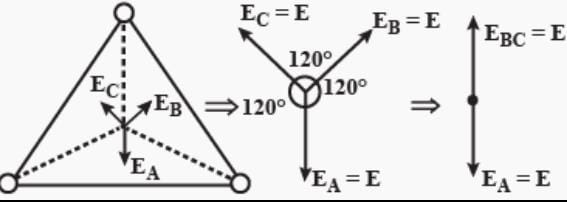JEE Main Mock - 3 (Previous Pattern) - Question 9

A lift of mass 1000 kg is moving with an acceleration of 1 m−s⁻2 in upward direction. Tension developed in the string, which is connected to the lift, is

Detailed Solution for JEE Main Mock - 3 (Previous Pattern) - Question 9 Use T-mg=ma with g=9.8You will get T=m(a+g)T=1000(1+9.8)T=1000(10.8)T=10800
JEE Main Mock - 3 (Previous Pattern) - Question 10

A machine gun fires a bullet of mass 40 g with a velocity 1200 m−s⁻1. The man holding it can exert a maximum force of 144 N on the gun. How many bullets can he fire per second at the most?

JEE Main Mock - 3 (Previous Pattern) - Question 11

A long solenoid has n turns per metre and current I ampere is flowing through it. The magnetic field at the ends of solenoid is

JEE Main Mock - 3 (Previous Pattern) - Question 12

The logic behind 'NOR' gate is that, it gives

JEE Main Mock - 3 (Previous Pattern) - Question 13

Two pieces of a glass plate, placed one upon the other with a little water in between them, can not be separated easily, because of

JEE Main Mock - 3 (Previous Pattern) - Question 14

The function of the moderator in a nuclear reactor is

JEE Main Mock - 3 (Previous Pattern) - Question 15

The sum of two vectors A → and B → is at right angles to their difference. Then

JEE Main Mock - 3 (Previous Pattern) - Question 16

In the following question, a Statement of Assertion (A) is given followed by a corresponding Reason (R) just below it. Read the Statements carefully and mark the correct answer-
Assertion(A):In a nuclear reactor cadmium acts as a moderator.
Reason(R):The cadium rods are so inserted in the graphite block so that they can be slided in and out as per requirement.

Detailed Solution for JEE Main Mock - 3 (Previous Pattern) - Question 16 The role of moderator is to slow down the neutron produced due to nuclear fission reaction occurring in nuclear reactors. The cadmium rod is good neutron absorber so it is used to control the number and not to slow them down for reuse.
JEE Main Mock - 3 (Previous Pattern) - Question 17

In the following question, a Statement of Assertion (A) is given followed by a corresponding Reason (R) just below it. Read the Statements carefully and mark the correct answer-
Assertion(A): Energy density in the solenoid is greater at centre than near its ends.
Reason(R): Energy density is defined as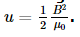JEE Main Mock - 3 (Previous Pattern) - Question 18

A solid cylinder of mass M and radius R rolls down an inclined plane without slipping. The speed of its centre of mass when it reaches the bottom is

Detailed Solution for JEE Main Mock - 3 (Previous Pattern) - Question 18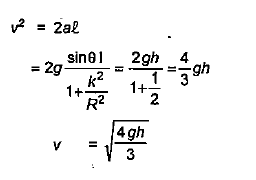JEE Main Mock - 3 (Previous Pattern) - Question 19

There are two lead spheres at the same temperature, the ratio of radii being 1:2 The ratio of head capacities are

JEE Main Mock - 3 (Previous Pattern) - Question 20

For the constructive interference the path difference between the two interfering waves must be equal to

JEE Main Mock - 3 (Previous Pattern) - Question 21

The fundamental unit which has the same power in the dimensional formula of surface tension and viscosity is

JEE Main Mock - 3 (Previous Pattern) - Question 22

If a star is moving towards earth, then the lines are shifted towards

Detailed Solution for JEE Main Mock - 3 (Previous Pattern) - Question 22 Its shifts towards blue because of increases in frequency by Doppler Effect in optics.
JEE Main Mock - 3 (Previous Pattern) - Question 23

A parallel monochromatic beam of light is incident normally on a narrow slit. A diffraction pattern is formed on a screen placed perpendicular to the direction of the incident beam. At the first minimum of the diffraction pattern, the phase difference between the rays coming from the two edges of slit is

JEE Main Mock - 3 (Previous Pattern) - Question 24

When a tuning fork of frequency 341 is sounded with another tuning fork, six beats per second are heard. When the second tuning fork is loaded with wax and sounded with the first tuning fork, the number of beats is two per second. The natural frequency of the second tuning fork is

JEE Main Mock - 3 (Previous Pattern) - Question 25

d/dx [tan-1 cosx/(1 + sin x)] =

Detailed Solution for JEE Main Mock - 3 (Previous Pattern) - Question 25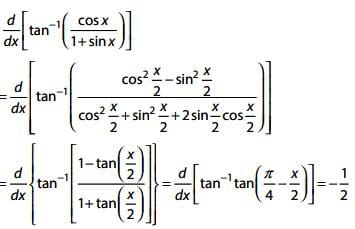JEE Main Mock - 3 (Previous Pattern) - Question 26

Singly ionized helium (He+) atom is hydrogen like in the sense that a solitary electron revolves around a positively charged nucleus. If the energy of this electron in its first orbit (n = 1) is -54.4 eV, what will be its energy in the first excited state?

JEE Main Mock - 3 (Previous Pattern) - Question 27

The charge deposited on 4 μ F capacitor in the circuit is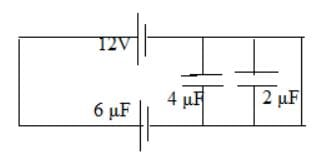JEE Main Mock - 3 (Previous Pattern) - Question 28

The angular velocity of the second needle in watch (in rad/s) is

JEE Main Mock - 3 (Previous Pattern) - Question 29

We are able to obtain fairly large currents in a conductor because

JEE Main Mock - 3 (Previous Pattern) - Question 30

A charge +4q is located on the X-axis of a Cartesian coordinate system at x = -2a as shown. Another charge +(q/4) is located on the X-axis at x = a. Where on the Y-axis should a third charge -3q be placed so that the resultant electric field at the origin O has equal positive X and Y components?

JEE Main Mock - 3 (Previous Pattern) - Question 31

A charged particle with velocity 2 x 103 m/s passes undeflected through electric and magnetic field. Magnetic field is 1.5 telsa. The electric field intensity would be

JEE Main Mock - 3 (Previous Pattern) - Question 32

The correct order of boiling points for primary (1º), secondary (2º) and tertiary (3º) alcohols is

JEE Main Mock - 3 (Previous Pattern) - Question 33

Which of the following does not undergo Cannizzaro's reaction?

JEE Main Mock - 3 (Previous Pattern) - Question 34

DNA has deoxyribose, base and a third compound, known as

JEE Main Mock - 3 (Previous Pattern) - Question 35

For reaction at equilibrium, Le Chatelier's principle predicts the effects of

JEE Main Mock - 3 (Previous Pattern) - Question 36

For an endothermic reaction, energy of activation is Ea and enthalpy of reaction is ΔH (both of these in kJ/mol). Minimum value of Ea will be

JEE Main Mock - 3 (Previous Pattern) - Question 37

In the reaction CH2COOHaq + OH(aq) → CH3COO- + H2O(l) ; ΔH = -55.2kJ mol-1 What is the heat of dissociation (in kJ mol-1) of acetic acid?

Detailed Solution for JEE Main Mock - 3 (Previous Pattern) - Question 37

ΔH = -55.2 KJ.mol-1
-[H+ (aq) + OH- (aq) → H2O(l) ΔH = -57.3 KJ .mol-1]]

CH2COOHaq → CH3COO- (aq) + H+ (aq) ΔH = ?
CH2COOH(aq) + OH- (aq) → CH3COO- (aq) + H2O(l) ΔH = -55.2 KJ .mol-1
-H+ + OH- (aq) → - H2O(l) ΔH = + 57.3KJ .mol-1
CH3COOH(aq) → CH3COO- (aq) + H+(aq) ΔH = 2.1 KJmol-1

JEE Main Mock - 3 (Previous Pattern) - Question 38

Element with Atomic number 38, belongs to

Detailed Solution for JEE Main Mock - 3 (Previous Pattern) - Question 38

Element with atomic number-38 is strontium (symbol-Sr), which belongs to II A group i.e. alkaline earth metals and 5th period.

JEE Main Mock - 3 (Previous Pattern) - Question 39

Which of the following complexes will have four isomers?

Detailed Solution for JEE Main Mock - 3 (Previous Pattern) - Question 39

The complex [Co(en)(NH3)2​Cl2​]Cl has four isomers. In one isomer, two ammonia ligands are trans to each other. In the other isomer, the chlorine ligands are trans to each other. In the third isomer the ammonia ligands are cis to each other and chlorine ligands are also cis to each other. This is optically active and exists in d and l forms. Thus, total four isomers are possible.

JEE Main Mock - 3 (Previous Pattern) - Question 40

Compound A has a molecular formula C₇H₇NO on treatment with Br₂/KOH, A gives an amine B which respond to carbylamine test. B on diazotisation and coupling with phenol give an azo dye. A can be

JEE Main Mock - 3 (Previous Pattern) - Question 41

The amount of Aluminium deposited when 0.1 Faraday current is passed through aluminium chloride will be (R = 27)

JEE Main Mock - 3 (Previous Pattern) - Question 42

A solution of AgNO3 during electrolysis between silver electrodes required a quantity of 241.25 coulombs of electricity the weight of silver deposited is

JEE Main Mock - 3 (Previous Pattern) - Question 43

pH of a solution is defined by the expression

JEE Main Mock - 3 (Previous Pattern) - Question 44

To a solution containing equimolar mixture of sodium acetate and acetic acid, some more amount of sodium acetate solution is added. The pH of mixture solution

JEE Main Mock - 3 (Previous Pattern) - Question 45

Identify the indicator used to titrate Na₂CO₃ solution with HCl

JEE Main Mock - 3 (Previous Pattern) - Question 46

A white salt is readily soluble in water and gives a colourless solutions with a pH of about 9.The salt would be

JEE Main Mock - 3 (Previous Pattern) - Question 47

Which of the following compounds will show positive silver mirror test?

JEE Main Mock - 3 (Previous Pattern) - Question 48

Among the following compounds the decreasing order of reactivity towards electrophilic substitution is: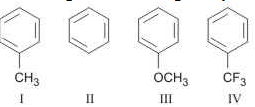JEE Main Mock - 3 (Previous Pattern) - Question 49

Which is not a macromolecule?

Detailed Solution for JEE Main Mock - 3 (Previous Pattern) - Question 49 The most common macromolecules in biochemistry are biopolymers (nucleic acids, proteins, carbohydrates and lipids) and large non-polymeric molecules (such as lipids and macrocycles).
JEE Main Mock - 3 (Previous Pattern) - Question 50

For preparing an alkane ,a concentrated aqueous solution of sodium or potassium salt of a saturated carboxylic acid is subjected to

JEE Main Mock - 3 (Previous Pattern) - Question 51

AB is an ionic solid. If the ratio of ionic radii of A+ and B is 0 . 52. What is the coordination number of B

Detailed Solution for JEE Main Mock - 3 (Previous Pattern) - Question 51

Ratio r + r - = 0.414 - 0.732
Coordination no. is 6.

JEE Main Mock - 3 (Previous Pattern) - Question 52

The solubility of gas in liquid increases with

JEE Main Mock - 3 (Previous Pattern) - Question 53

Which of the following is not a crystalline solid ?

JEE Main Mock - 3 (Previous Pattern) - Question 54

A 100 g solution contains 25 g of water, 25 g ethanol and 50 g acetic acid. The mole fraction of water is

JEE Main Mock - 3 (Previous Pattern) - Question 55

Pent-1-ene with HCl gives

JEE Main Mock - 3 (Previous Pattern) - Question 56

Which of the following statements is incorrect?

Detailed Solution for JEE Main Mock - 3 (Previous Pattern) - Question 56

No two spectral lines of an element can have the same wave number.

JEE Main Mock - 3 (Previous Pattern) - Question 57

Entropy of universe:

Detailed Solution for JEE Main Mock - 3 (Previous Pattern) - Question 57 Entropy means randomness in accommodation of internal energy of gas and the randomness can only increase because in order to cool down a place we need the throw the heat energy somewhere else so randomness decreases at one point but increases at other point. overall it increases only. there since universe is an isolated system (assumed) it will always a increase in randomness of the universe.
JEE Main Mock - 3 (Previous Pattern) - Question 58

An activated complex molecule differs from a normal molecule of the same atomicity in that it has__

JEE Main Mock - 3 (Previous Pattern) - Question 59

The electrons, identified by quantum numbers n,l (i) n = 4, l = 1, (ii) n = 4, l = 0, (iii) n = 3, l = 2, (iv) n = 3, l = 1 can be placed in order of increasing energy, from the lowest to highest, as_

Detailed Solution for JEE Main Mock - 3 (Previous Pattern) - Question 59

On the basis of (n + l) rule, the values for (i), (ii), (iii) and (iv) are 5,4,5 and 4 respectively. In these in both, but n is minimum for (iv). Hence energy order = (iv) > (ii). Similarly in (i) and (ii), hence (iii) < (i).

JEE Main Mock - 3 (Previous Pattern) - Question 60

Which one of the following platinum complexes is used in cancer chemotherapy?

Detailed Solution for JEE Main Mock - 3 (Previous Pattern) - Question 60

Cis isomer of [PtCl2(NH3)2] is known as cisplatin and is used as an antitumor agent in treatment of cancer.

JEE Main Mock - 3 (Previous Pattern) - Question 61

If 0.6387 of plantinichloride of a monoacid base on ignition gave 0.209g of platinum, then molecular weight of the base is

JEE Main Mock - 3 (Previous Pattern) - Question 62

The area, in square unit, of the region bounded by the curve x2 = 4 y , the line x = 2 and the x-axis is

JEE Main Mock - 3 (Previous Pattern) - Question 63

When 599 is divided by 13 then the remainder is

Detailed Solution for JEE Main Mock - 3 (Previous Pattern) - Question 63
Rem [5^99/13] = 8

We need to find out the remainder when 5^99 is divided by 13

We should try and reduce the numerator in this case. Let us have a look at the method below.

Remainder [5^99 / 13]

= Remainder [5*5^98 / 13]

= Remainder [5*25^49 / 13]

= Remainder [5*(-1)^49 / 13]

= Remainder [(-5) / 13]

= 8
JEE Main Mock - 3 (Previous Pattern) - Question 64

What is the value of x satisfying the equation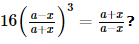?

JEE Main Mock - 3 (Previous Pattern) - Question 65

The equation of the circle described on the common chord of the circles x2 + y2 -8x + y -15 = 0 and x2 + y2 - 4x + 4y - 42 = 0 as diameter is

JEE Main Mock - 3 (Previous Pattern) - Question 66

If p and q are the roots of the equation x2 + px + q = 0, then

Detailed Solution for JEE Main Mock - 3 (Previous Pattern) - Question 66

p + q = -p and pq = q => q(p - 1) = 0 => q = 0 or p = 1.
If q = 0, then p = 0. i.e., p – q \ p = 1 and q = -2.

JEE Main Mock - 3 (Previous Pattern) - Question 67

i243 =

JEE Main Mock - 3 (Previous Pattern) - Question 68

If z lies on |z| = 1 ,then 2/z lies on

Detailed Solution for JEE Main Mock - 3 (Previous Pattern) - Question 68 Let z=x+iy

so |z|=1. => x²+y²=1

Let 2/z = another complex no. c

c= 2/z
= 2/(x+iy)
= 2(x-iy)/(x²+y²)
= 2(x-iy)

so |c| = 2|x-iy|
= 2(x²+y²)
= 2

so |c|= 2. which represents a circle
JEE Main Mock - 3 (Previous Pattern) - Question 69

The solution of the equation y - x(dy/dx)=a[(y2)+(dy/dx)] is

JEE Main Mock - 3 (Previous Pattern) - Question 70

"If Deb and Sam go to the mall, then it is snowing."
Which statement below is logically equivalent?

Detailed Solution for JEE Main Mock - 3 (Previous Pattern) - Question 70

A statement is logically equivalent to its contrapositive.

JEE Main Mock - 3 (Previous Pattern) - Question 71

In the following question, a Statement of Assertion (A) is given followed by a corresponding Reason (R) just below it. Read the Statements carefully and mark the correct answer-
A committee of n persons is to be formed out of 30 persons.
Assertion(A) : The value of n for which the number of committees is maximum is n = 15
Reason(R) : In the expansion of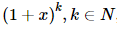the middle term has the greatest binomial coefficient.

JEE Main Mock - 3 (Previous Pattern) - Question 72

In the following question, a Statement of Assertion (A) is given followed by a corresponding Reason (R) just below it. Read the Statements carefully and mark the correct answer-
Assertion(A):The angle between the lines 2x = 3y = -z and 6x = - y = - 4z , is 900 .
Reason(R): If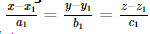andand angle between them is θ , then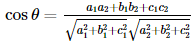JEE Main Mock - 3 (Previous Pattern) - Question 73

If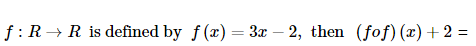JEE Main Mock - 3 (Previous Pattern) - Question 74

A and B are square matrices of order n x n, then (A - B)2 is equal to

Detailed Solution for JEE Main Mock - 3 (Previous Pattern) - Question 74

(A - B) x (A - B)
(A - B) xA - (A - B) x B
= A2 - AB - BA + B2

JEE Main Mock - 3 (Previous Pattern) - Question 75

The equation of the normal to the curve x2 = 4y at (1, 2) is

JEE Main Mock - 3 (Previous Pattern) - Question 76

If 2nC3:nC2=44:3.For which value of r, nCr=15?

JEE Main Mock - 3 (Previous Pattern) - Question 77

If a dice is thrown twice, the probability of occurrence of 4 at least once, is

Detailed Solution for JEE Main Mock - 3 (Previous Pattern) - Question 77 N(s)=6^2=36n(occurrence of 4 atleast once)={ (1,4),(2,4),(3,4),(4,4),(5,4),(6,4),(4,1),(4,2),(4,3),(4,5),(4,6)}=11P(occurrence of 4 atleast once)=n(occurrence of 4 atleast once)/n(s)=11/36
JEE Main Mock - 3 (Previous Pattern) - Question 78

A student obtain 75%, 80% and 85% in three subjects. If the marks of another subject are added, then his average cannot be less than

JEE Main Mock - 3 (Previous Pattern) - Question 79

The angle between lines 3x+y-7=0 and x+2y+9=0 is

Detailed Solution for JEE Main Mock - 3 (Previous Pattern) - Question 79 Use the formula tan thita=|(m1-m2)/1+m1m2|
JEE Main Mock - 3 (Previous Pattern) - Question 80

The equation of the sphere passing thro' the point (1,3-2) and the circle y2+z2=25 and x=0 is

JEE Main Mock - 3 (Previous Pattern) - Question 81

If (axb)2+(a.b)2=144 and |a|=4, then |b|=

JEE Main Mock - 3 (Previous Pattern) - Question 82

Given that θ is a acute and that sin θ =3/5. Let x,y be positive real numbers such that 3(x-y) =1, then one set of solutions for x and y expressed in terms of θ is given by

JEE Main Mock - 3 (Previous Pattern) - Question 83

The length of subtangent to x2 + xy + y2 = 7 at 1, − 3 is

JEE Main Mock - 3 (Previous Pattern) - Question 84

If a=i+2j-2k, b=2i-j+k, c=i+3j-k, then ax(bxc)=

JEE Main Mock - 3 (Previous Pattern) - Question 85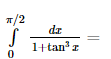JEE Main Mock - 3 (Previous Pattern) - Question 86

A and B are non - zero square matrices of the same order. Which is correct?

Detailed Solution for JEE Main Mock - 3 (Previous Pattern) - Question 86

From the properties of the matrices, if A,B are non-zero square matrices of same order such that AB=0 then the either of the matrices must be singular matrix.

A singular matrix is a matrix whose determinant is zero.

Therefore, ∣A∣=0 or ∣B∣=0

JEE Main Mock - 3 (Previous Pattern) - Question 87

In the following question, a Statement of Assertion (A) is given followed by a corresponding Reason (R) just below it. Read the Statements carefully and mark the correct answer-
Assertion(A):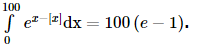Reason(R): If f(x) is a periodic function of period a, then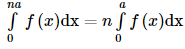JEE Main Mock - 3 (Previous Pattern) - Question 88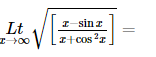JEE Main Mock - 3 (Previous Pattern) - Question 89

If minimum value of f(x) = x2 + 2bx + 2c2 is greater than maximum value of g(x) = - x2 - 2cx + b2 then for x is real

JEE Main Mock - 3 (Previous Pattern) - Question 90

Let f(x) =ax2+bx+c,a≠0 and triangle = b2-4ac.If α+β, α22 and α22 are in G.P., then :

Detailed Solution for JEE Main Mock - 3 (Previous Pattern) - Question 90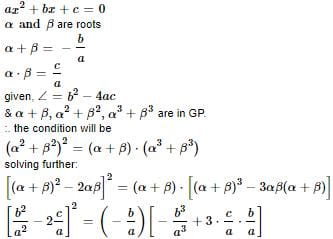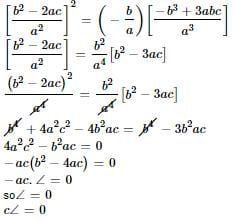## Mock Test Series for JEE Main & Advanced

2 videos|324 docs|160 tests
 Use Code STAYHOME200 and get INR 200 additional OFF Use Coupon Code
Information about JEE Main Mock - 3 (Previous Pattern) Page
In this test you can find the Exam questions for JEE Main Mock - 3 (Previous Pattern) solved & explained in the simplest way possible. Besides giving Questions and answers for JEE Main Mock - 3 (Previous Pattern), EduRev gives you an ample number of Online tests for practice

## Mock Test Series for JEE Main & Advanced

2 videos|324 docs|160 tests